# Team:British Columbia/Consortia

British Columbia - 2012.igem.org

Modeling Microbial Consortia: The Auxotroph Approach

There is a large potential combinatorial space for optimizing and tuning a three-member microbial consortium with auxotrophic interdependence. In order to help address this concern, we set to develop a computational framework to help guide experimental decisions. Our model attempts to develop a predictive understanding of auxotrophic interdependence in a three-member microbial consortium. As it was important for us to be able to predict growth kinetics based on measurable, empirical properties, as well as to develop a mathematically and experimentally tractable system, we decided to base our model on Monod kinetics.

The Monod equation, models the growth of microorganisms in aqueous environments. The underlying assumption is that the growth rates are dependent on the concentration of a limiting nutrient. This equation relates the specific growth rate (µg, the specific growth rate of microorganisms) to the maximum growth rate (µm), the saturation constant (or half-velocity constant, Ks) as a function of the concentration of the limiting substrate (S) .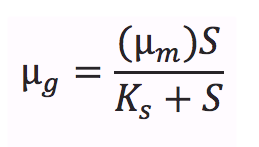The three interdependent E. coli auxotrophs used in our project are ΔtrpA, ΔmetA, and ΔtyrA. Each of these strains is missing a gene critical in the respective amino acid biosynthetic pathway. Each of these amino acids are treated as the growth limiting substrates. The model is extends on the work recently presented by Kerner and colleagues  and attempts to predict the growth and survival of a three-member consortia.

It takes into account a number of factors:
1. The constitutive production of each required amino acid by the two remaining natural prototrophs
2. The inducible production of each required amino acid by a recombinant prototroph, and
3. The uptake of amino acids in each auxotroph.

The following sections detail the mathematical foundation of the model, the experimental measurement of the necessary constants, the MATLAB implementation and some preliminary results.

Equations and Constants

We proposed the following fundamental equations for our consortium model. In this model, each auxotrophic strain is denoted with a superscript minus (i.e. ΔtrpA, ΔmetA, and ΔtyrA are denoted trpA-, metA-, and tryA-, respectively); [Trp], [Met], and [Tyr] represent the concentrations of tryptophan, methionine, and tyrosine; ODtrpA-, ODmetA-, and ODtyrA- represent the optical densities of the ΔtrpA, ΔmetA, and ΔtyrA strains; a1, a2, and a3 are the consumption constants with respect to changes in ODtrpA-, ODmetA-, and ODtyrA-, respectively; µtrpA-, µmetA-, µtyrA- are the specific growth rates of the ΔtrpA, ΔmetA, and ΔtyrA strains; µMaxtrpA-, µMaxmetA-, µMaxtyrA- are the maximum growth rates of the ΔtrpA, ΔmetA, and ΔtyrA strains; and KstrpA-, KsmetA-, KStyrA- are the half-velocity constants of the ΔtrpA, ΔmetA, and ΔtyrA strains. The terms and constants of this equation will be discussed in further detail later.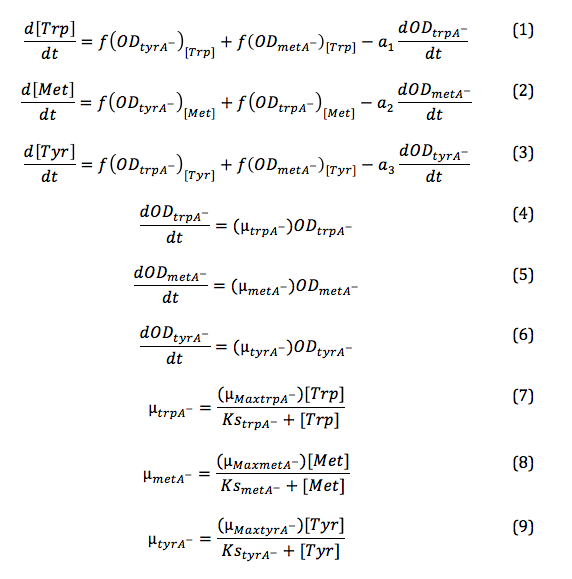Please note that: equation (7), (8), and (9) can be substituted into equation (4), (5), and (6) directly, and equation (4), (5), (6) after the substitution can be further substituted into equation (1), (2), and (3) to simplify the code further. The overall three equations shown later in the Matlab Code section is based on the described substitution. It is indicated here for viewers' convenience of following the Matlab code later.

In the above equations, some of the key constants for the Monod Kinetics are obtained through analyzing the growth curves in a 96-well plate, with varying amino acid concentrations. Characteristic growth rates were observed and plotted:

Applying the Monod kinetic model, we sought out to measure three variables: the concentration of tryptophan, methionine, and tyrosine in the media available for each specific auxotroph. We were also interested in how these concentrations vary with respect to time.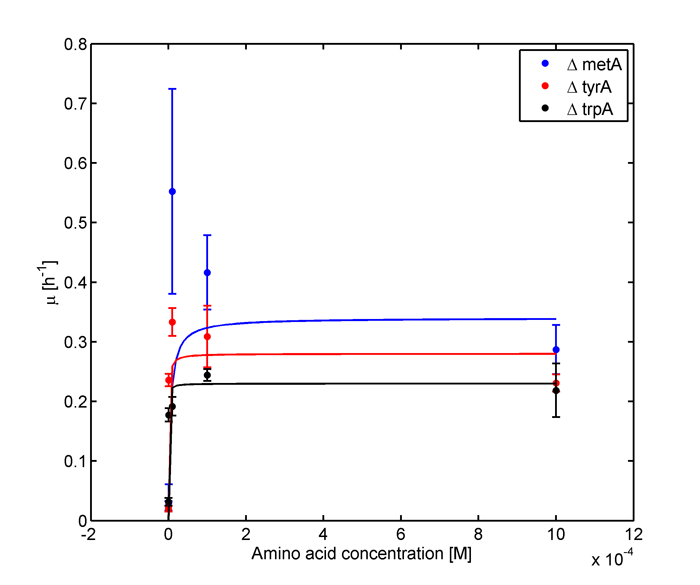Figure 1: Monoculture Growth Rates at various Limiting Amino Acid Concentrations.

These data are fit to the Monod equation to determine the constants listed below.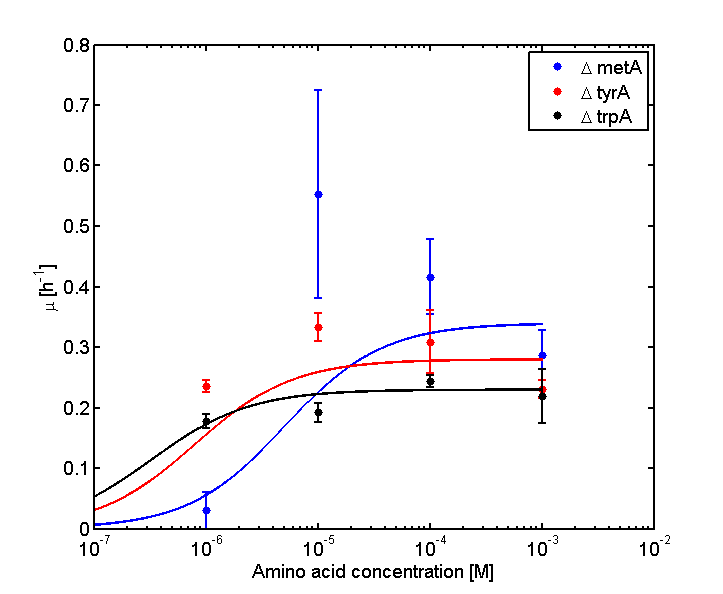Figure 2: Log Scale of Monoculture Growth Rates at various Limiting Amino Acid Concentrations.

Note: we used lower amino acid concentrations as well, however we found out that when concentration is below 1E-7 M, we observed no significant overnight growth of the cells. For a detailed protocol, please visit here.

Based on the fits presented in Figures 1 and 2, the key constants, such as maximum growth rates, and half-velocity constants, for each auxotroph were obtained :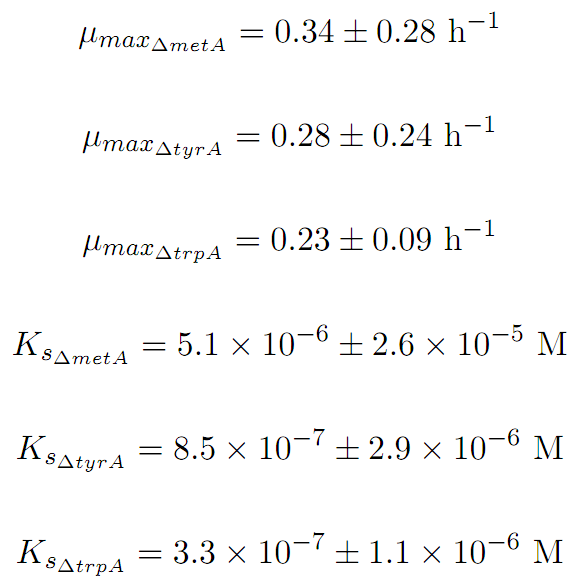As discussed in the beginning of this section, we assumed that the amino acid consumption is solely dependent on the cell growth rate. In order to find out the consumption rate constants, (a1, a2, and a3), we need to find out a way to measure the amino acid concentrations in the media with respect to the change of its OD for each auxotroph. To do this, we need to find a way to measure media amino acid concentrations. With no good access to HPLC, we noticed in previous experiments that each of the knockout monocultures will reach to a certain final OD at a different known concentration of amino acid. In addition, recently published paper: ''A Programmable Escherichia coli Consortium via Tunable Symbiosis'', Kerner A et. al (2012) confirmed that there is indeed a relationship between the final auxotroph ODs with the environmental amino acid concentrations . We thus generated a calibration curve to relate the limiting amino acid concentrations to their final OD readings. We based this on our own wet lab data shown in the figures below: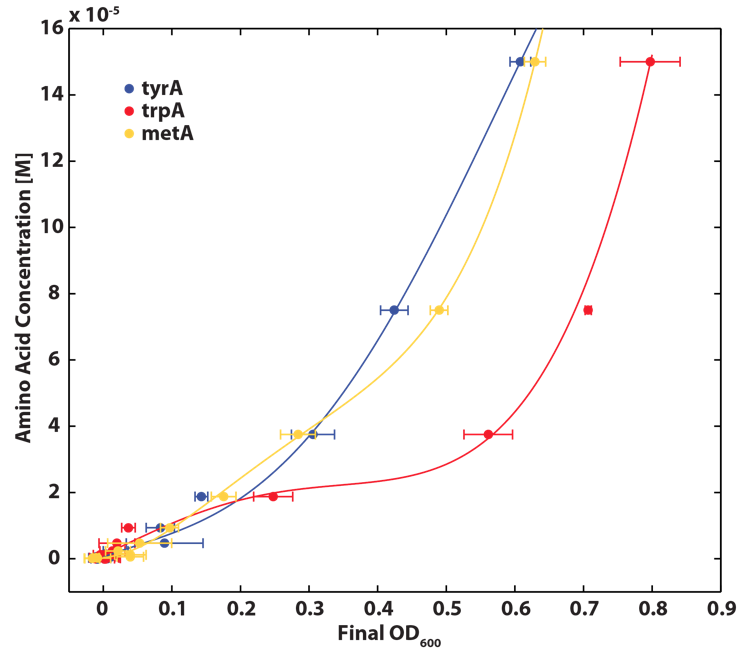Figure 3: Calibration curves used to convert final OD600 to initial amino acid concentration. Standard solutions of known amino acid concentrations were innoculated with the respective auxotroph and grown for 20 hours at 37oC shaken at 200RPM, after which final OD600 were measured. Data were fit to quartic polynomials to inter- polate OD600 readings. Error bars represent standard deviation (n=3).

.

We then sought out to find out how the amino acid in the environment is consumed with respect to cell growth.

The three auxotrophs were grown in 50 ml flasks with known initial Tryptophan, Methionine, and Tyrosine concentrations (5E-5 M), respectively, and 5ml of cell free supernatants at various ODs was isolated. These supernatants served as new 96-well plate media for each knock out cultures with newly added M9-Glucose shots (2% glucose in the well) to ensure the amino acids of interest remained as the limiting substrate for all knock outs. A detailed protocol can be found here.

The cells were grown in a 96-well plate for 15 hours at 37 oC, and growth curve data was obtained using a plate reader (most of the monocultures reached stationary phase at this point).

Based on the calibration curves (Figure 3), the amino acid concentrations in the wells were calculated from the final OD readings, and when corrected with a dilution factor of 5/4 (as we added in the 5X, it was 1/5 of the total well media volume, as described here), we obtained the resultant amino acid concentrations in the supernatant at various auxotroph monoculture densities. Again, with the assumption that the only change in the amino acid concentration in the media is due to cell growth, and as they are specific knock outs, they were not able to release any amino acid into the environment, each auxotrophs' consumption constants with respect to cell growth rate (a1, a2, a3) can be calculated. Their values are as follows: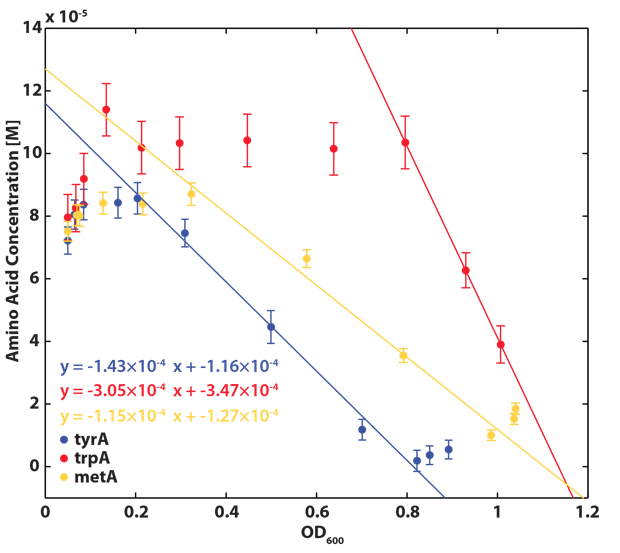Figure 4. Amino acid consumption measured from auxotroph cultures in minimial media spiked with an initial concentration of 0.15 mM of the required amino acid. Linear regression was used to find the following consumption rates: Trp: 0.304 ± 0.009 mM/OD (a1), Met: 0.115 ± 0.047 mM/OD (a2), Tyr: 0.143 ± 0.020 mM/OD (a3). Error bars represent 95% confidence interval as determined by regression in the calibration curves.

Now that we have our consupmtion constants, we were interested in finding out how the other two auxotrophs will contribute to changes in environmental amino acid concentrations.

With regards to the behaviour of auxotrophs in coculture under changing amino acid concentrations, the closest assumption we can make is that each auxotroph will behave similarly to the conditions of monoculture. Thus, we used the same calibration curve for relating the final OD to the limiting substrate amino acid concentration in the media. The difference here is that, instead of finding out the corresponding limiting amino acid concentration, e.g., ΔmetA media for [Met] measurements, we are interested in measuring the other two non-limiting amino acid concentrations of each knocked out E. coli auxotrophs, e.g. ΔmetA media for [Trp] and [Tyr] concentrations, at various OD readings . We were then able to derive six functions that relate the change of all amino acid concentrations with respect to time (e.g. d[Trp]/dt), based on two auxotrophs' optical densities (e.g. ODmetA- and ODtyrA-), as shown below: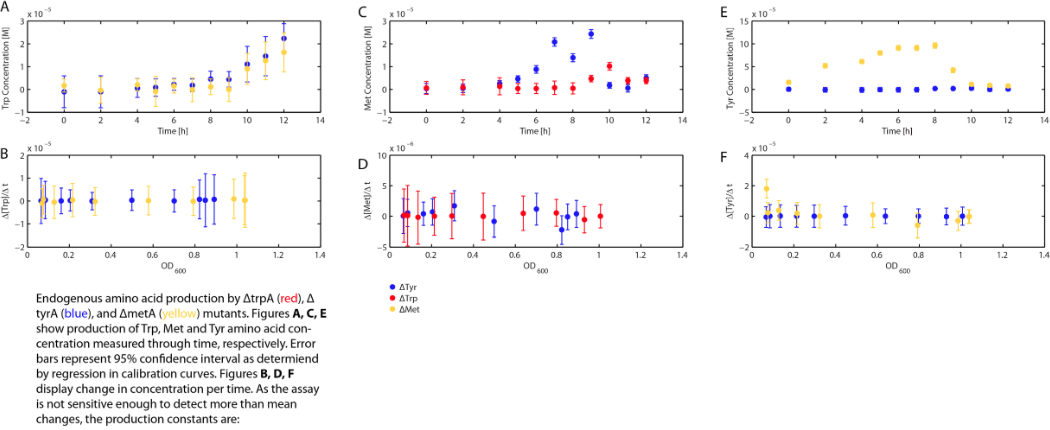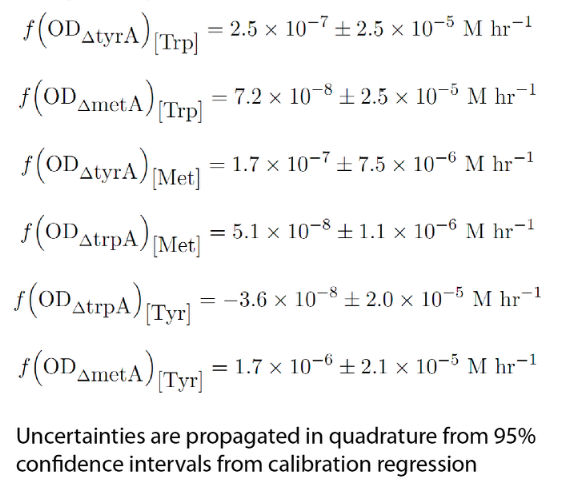Note: These equations can now be directly substituted into equations (1), (2), and (3), in order to simplify the Matlab Code section.

One shortcoming in the use of these equations is their dependency on the accuracy of the calibration curves (Figure 3, 4, and 5), as well as the OD readings, especially when OD readings are low and noisy (< 0.1, nearing the limits of the plate reader's accuracy).

For example, one of the experimental raw data obtained are shown below: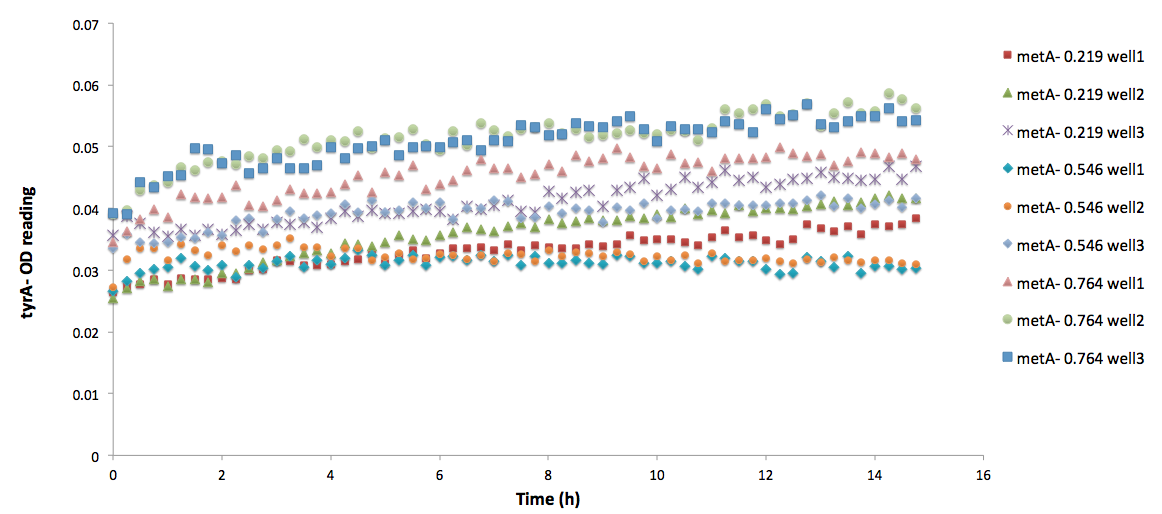Figure 5: ΔtyrA auxotroph growth curve in various ΔmetA ODs cell free supernatants in 96-well plate reader environment.

.

Note: As discussed before, this may lead to inaccuracies in calculation from low OD readings. All data sets are labelled correspondingly, and triplicate measurements are noted by wells 1, 2, and 3. As we can see, there are some distinct differences in the OD readings among the three different supernatant data sets; however, when corrected by focusing on the difference between the initial reading and the final reading with a base adjustment of 0.025 (the lower value used for all the calibration curves), they average roughly the same, with an average OD of 0.03847, 0.04043, and 0.04218, respectively, for ODmet 0.219, 0.546, and 0.764.

To illustrate this point more clearly, here is a comparison with another set of growth curve data run in the same plate.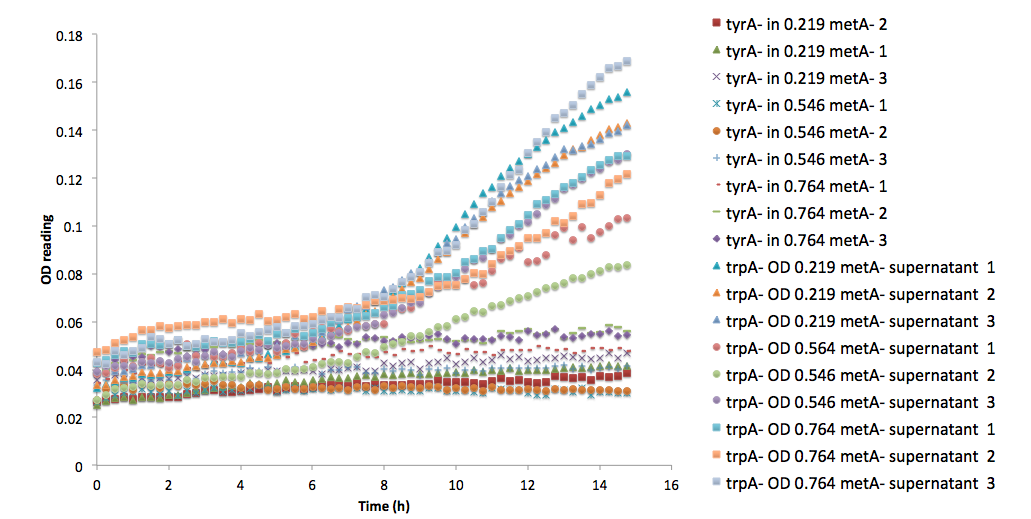Figure 6: Comparison between ΔtrpA and ΔtyrA auxotroph growth curve in the same supernatants of ΔmetA at its various optical density media.

Note that all three of the trpA- sets of growth curve grew to a much higher OD (reaching about 0.17) at the end of 15 hours and did not reach stationary phase, whereas all tyrA- sets grew significantly less, no significant change in OD at the end of the experiment.

Matlab Code

Now with all the key functions and constants approximated, we are able to encode our model into Matlab, where the six key ODE functions can be solved and output the our prediction of population dynamics.

The code is shown below, which contains a detailed step by step instruction for future iGEM teams or related scientific researches use, as well as the clarifications of our own code. It is also written is a way that it can be directly copied and pasted into Matlab blank m.files with all the comments in Matlab commenting format already, so that the coder can read the coded instructions and explanations in the coding command window without having to switching between, for example, the matlab and browser windows.

Of course, copying and pasting the code here is welcomed!

Code begins here:

% input the following commands, which are all in bold, into MATLAB command window, and not the m.file, before you call the ODE function.

%firstly, define a time array, set the upper limit to be the time (hr) you want this model to predict. For this model to be accurate, it is advised not to use extreme values.

%in our cases, the upper limit is set to 12 as some of the modelling equations are based on 11 hour culture supernatant data. Thus accuracy is expected within 12 hours.

% xspan = [0 12];

%in order to set up the initial conditions, where they corespond to the initial values of y(1), y(2), y(3)..., to y(6) in our case, and y(n) if you have n number of ODE equations

% ynot = [4E-5 4E-5 4E-5 0.05 0.05 0.05]';

% the first three values are the initial values for y(1), y(2), and y(3) respectively, which in out cases are the initial amino acid concentrations, and as we are adding the cells directly into the M9 media without supplementing any amino acids, it is believed to be at a low concentrations, though there will be some left over amino acids within the living initial cells, and thus is set to 4E-5 mole/L.

% the remaining three values are the initial conditions for y(4), y(5), and y(6), which are the initial cell ODs in out case, and they are all set to 0.05, which is the initial OD reading we obtained after we innoculated the culture, with the assumption that all the cells are still alive.

%Then RUN THE FUNCTION

% [X,Y] = ode45(@UBCiGEM2012_ConsortiaModel,xspan,ynot);

% this takes the function name in your m.file, the range of your interest, and the initial conditions which is just defined, respectively

%Note: have to make sure that the name is exactly the same as the function name in your m.file for it to be able to run correctly.

%the following code is for the actual mfile.

% 1) Declare the name of the function:

function dy = UBCiGEM2012_ConsortiaModel(t,y);

% 2) define all your ODE variables clearly for your own understanding as they can get quite confusing if you don't.

% in our case:

% y(1) = [Trp];

% dy(1) = d[Trp]/dt;

% y(2) = [Met];

% dy(2) = d[Met]/dt;

% y(3) = [Tyr];

% dy(3) = d[Tyr]/dt;

% y(4) = [OD_trpA-];

% dy(4) = d[OD_trpA-]/dt;

% y(5) = [OD_tyrA-];

% dy(5) = d[OD_tyrA-]/dt;

% y(6) = [OD_metA-];

% dy(6) = d[OD_metA-]/dt;

% 3) define your constants first that will not depend on variables

% in our case, it was all measured through our own wet lab data and is explained in the previous section

%define maximum growth rate constants

u_maxtrpA = 0.23; % units = hr-1

u_maxmetA = 0.34; % units = hr-1

u_maxtyrA = 0.28; % units = hr-1

%define half-velocity constants

Ks_trpA = 3.30E-7; % units = M

Ks_metA = 5.10E-6; % units = M

Ks_tyrA = 8.50E-7; % units = M

%define the consumption rate constants

a1 = 3.05E-4; %units= M/OD trp

a2 = 1.15E-4; %units= M/OD met

a3 = 1.4E-4; %units= M/OD tyr

% 4) write out ODE CODE, suggest to write out all equations on paper first, as they are a lot more clear than coding them directly, and will reduce the chance for you to make error why coding significantly.

dy = zeros(6,1);

dy(1) = 2.5E-7 + 7.2E-8 - a1*(u_maxtrpA*(y(1))/(Ks_trpA+y(1)))*y(4);

dy(2) = 1.7E-7 + 5.1E-8 - a2*(u_maxmetA*(y(2))/(Ks_metA+y(2)))*y(5);

dy(3) = -3.6E-8 + 1.7E-6 - a3*(u_maxtyrA*(y(3))/(Ks_tyrA+y(3)))*y(6);

dy(4) = (u_maxtrpA*y(1))/(Ks_trpA+y(1))*(y(4));

dy(5) = (u_maxmetA*y(2))/(Ks_metA+y(2))*(y(5));

dy(6) = (u_maxtyrA*y(3))/(Ks_tyrA+y(3))*(y(6));

% note: For easy coding and checking purposes, all this equations are exactly the same as the one shown in equation (1), (2), and (3) for dy(1), dy(2), and dy(3) respectively, with also the same arrangements of each equation term within them.

% and Please also note: these equation has been derived with direct simple substitution of equations, where equation (4), (5), (6), (7), (8), (9), (10), (11), (12), (13), (14) and (15) have been substituted into the equation (1), (2) and (3).

%if you want, you can choose to plot diagrams and display the predicting dynamics within the m.file or manually plot the arrays of your interests in command window

%For example, for this project, we are interesting in predicting the ODs of each auxotrophs, thus, we manually plotted values in y(4), y(5), and y(6) (which again, are the OD values of each auxotrophs) agaisnt time, by typing the following in the command window after run the ODE45 functions:

%>>plot(X,Y(:,4:6))

End of the m.file

Simulation Results, Analysis and Future Work

When the Matlab Code was run, it generated a graphic result predicting the population dynamics without inducing, shown below: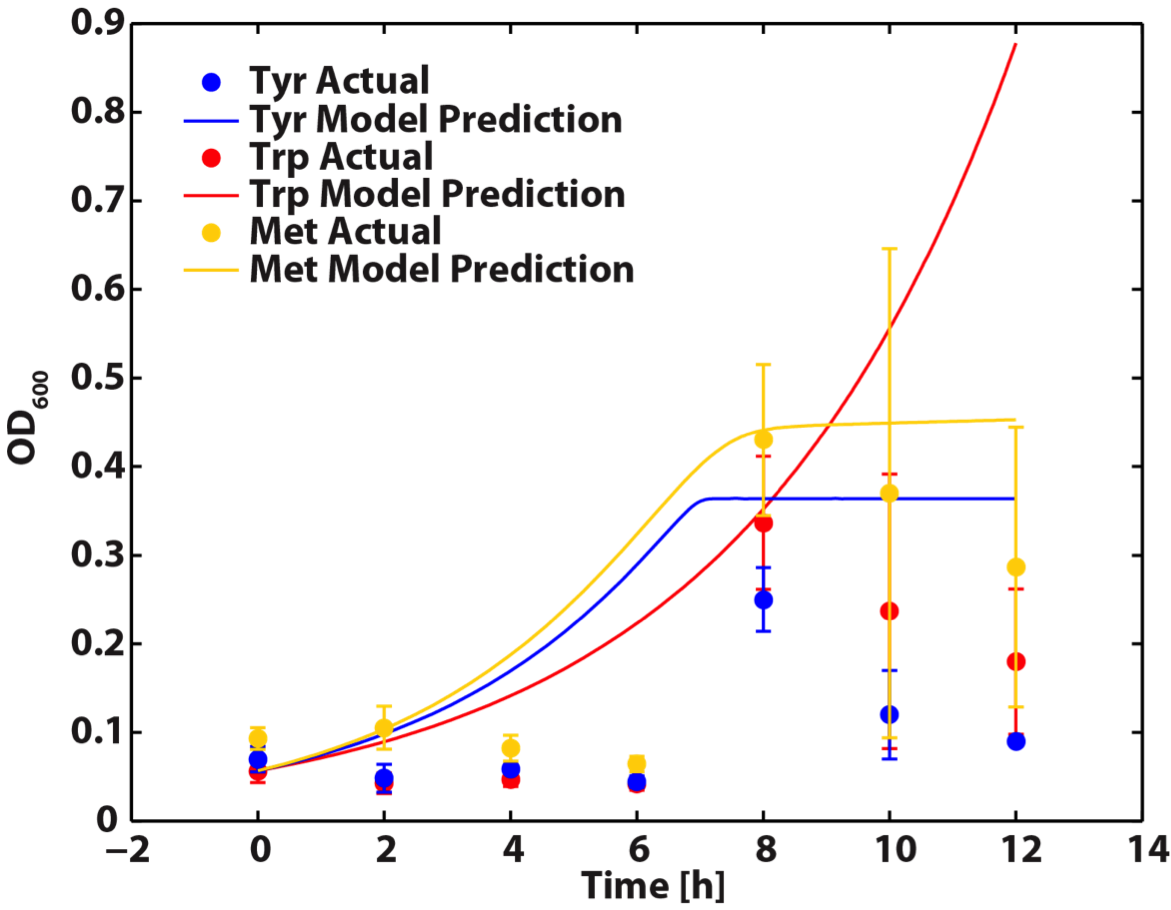Figure 7. A comparison of model predictions versus empirical measurements (n=3) of each auxotroph’s growth in a co-culture.

As shown in the figure above, this model can give reasonable predictions though still can be further modified for better accuracy. For our future work, we will refine our model with more experimental data. This can be accomplished by running the amino acid experiment data points of interest. Alternatively, we can use more direct methods of amino acid measurement, like HPLC analysis.

This code can also be adapted to predict the population dynamics of our cocultures when tuning effects are introduced. This is accomplished by by generating six new functions to describe the amino acid dynamics of each monoculture. We can then use this model to decide the amount of tuning (by adding in different amount of inducer) needed to obtain a desired population of each auxotroph.

References

 Shuler, ML; Kargi, F; Bioprocess Engineering Basic Concepts, 2002 2nd edition. Prentice Hall PTR.

 Kerner A; Park J; Williams A; Lin XN; A Programmable Escherichia coli Consortium via Tunable Symbiosis, 2012. PLoS ONE 7(3): e34032. doi:10.1371/journal.pone.0034032